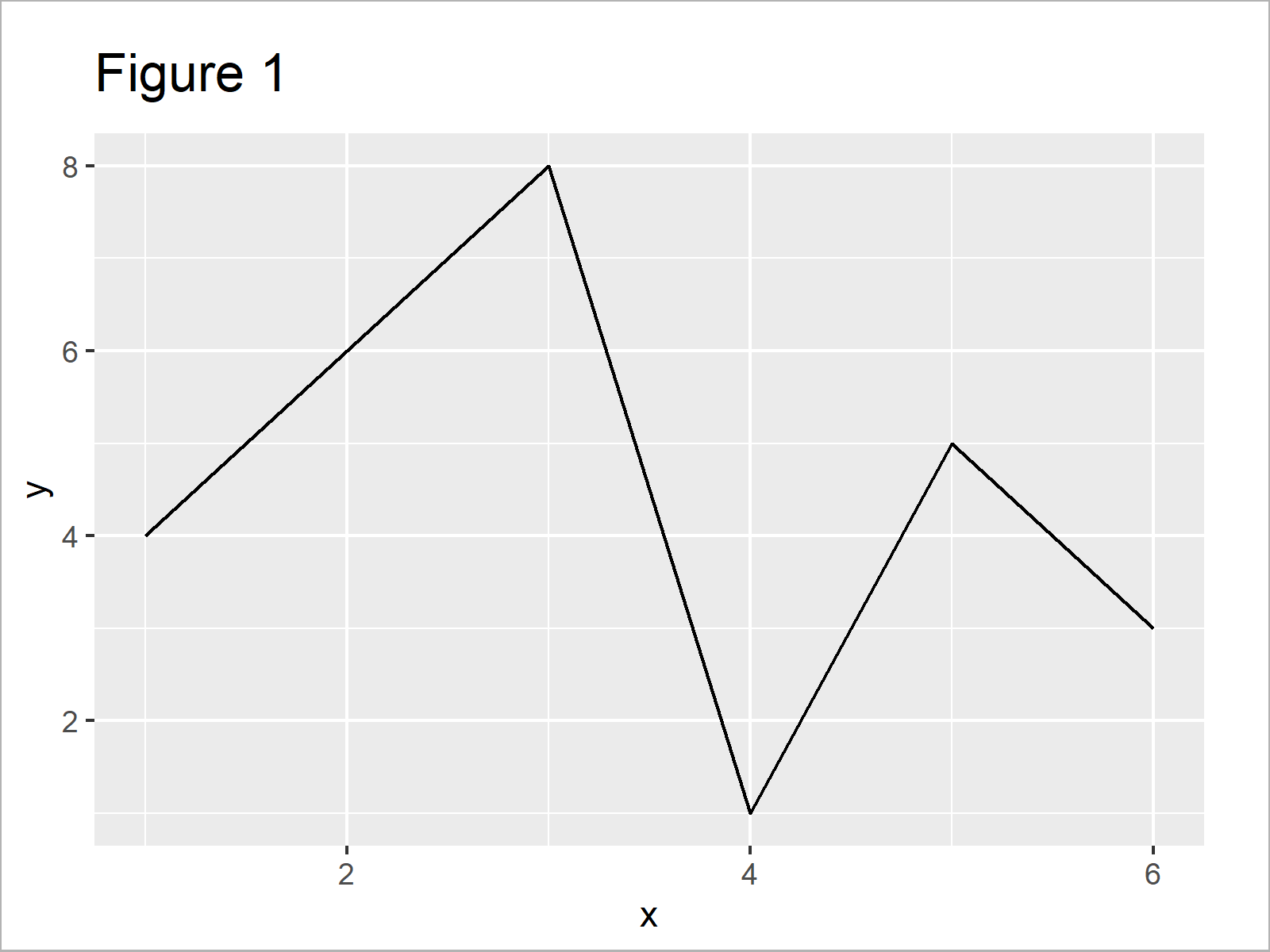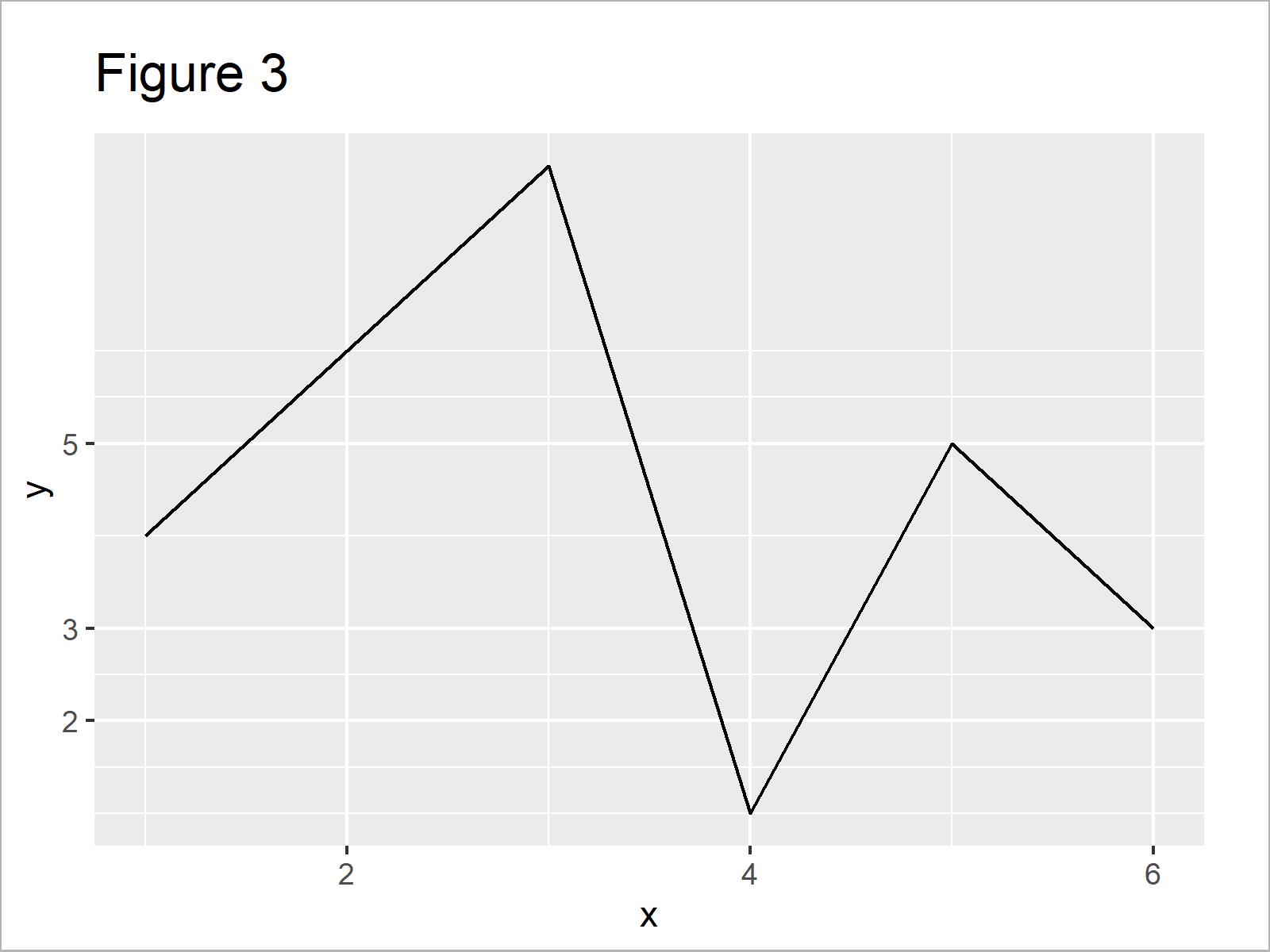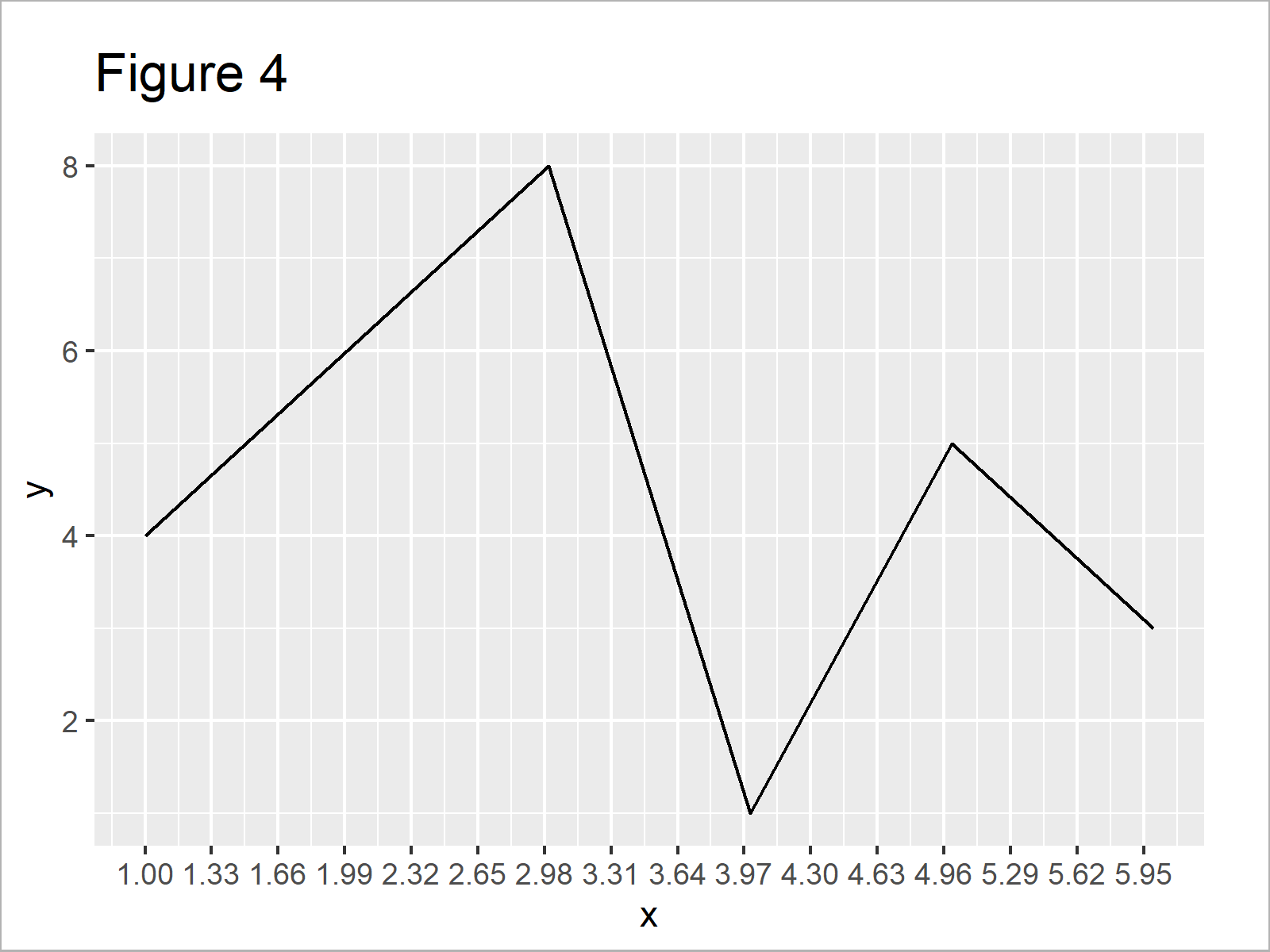# Set Axis Breaks of ggplot2 Plot in R (3 Examples)

In this R programming tutorial you’ll learn how to change the axis tick marks of a ggplot2 graphic.

The tutorial will consist of the following content:

It’s time to dive into the examples:

## Exemplifying Data, Add-On Packages & Basic Graphic

The first step is to create some example data:

```data <- data.frame(x = 1:6,         # Create example data
y = c(4, 6, 8, 1, 5, 3))
data                                # Print example data```Have a look at the table that got returned by the previous syntax. It illustrates that our example data is made of six rows and two columns.

In this R code, we also have to install and load the ggplot2 package:

```install.packages("ggplot2")         # Install & load ggplot2 package
library("ggplot2")```

As next step, we can draw a graph of our data:

```ggp <- ggplot(data, aes(x, y)) +    # Create default ggplot2 plot
geom_line()
ggp                                 # Draw default ggplot2 plot```Figure 1 shows the output of the previous code – A ggplot2 line plot with default axis ticks.

## Example 1: Manually Specify X-Axis Ticks in ggplot2 Plot

The following syntax illustrates how to adjust the axis tick positions of a ggplot2 plot using the scale_x_continuous function and the breaks argument.

Have a look at the following R code:

```ggp +                               # Manually specify x-axis ticks
scale_x_continuous(breaks = c(2, 3, 5))```The output of the previous R syntax is shown in Figure 2: A ggplot2 line chart with manual axis ticks on the x-axis.

## Example 2: Manually Specify Y-Axis Ticks in ggplot2 Plot

The following code illustrates how to set the axis breaks of a ggplot2 plot on the y-axis.

For this, we can basically use the same code as in Example 1. We simply need to replace the scale_x_continuous function by the scale_y_continuous function:

```ggp +                               # Manually specify y-axis ticks
scale_y_continuous(breaks = c(2, 3, 5))```Figure 3 shows the output of the previous R code – A ggplot2 graphic with user-defined axis values on the y-axis.

## Example 3: Manually Specify Sequence of Axis Ticks in ggplot2 Plot

In Example 3, I’ll illustrate how to create a user-defined sequence of axis breaks.

For this task, we can apply the seq function within the scale_x_continuous function as shown below:

```ggp +                               # Manually specify sequence of x-axis ticks
scale_x_continuous(breaks = seq(1, 6, 0.33))```The output of the previous R code is shown in Figure 4 – A ggplot2 graph with a manually specified sequence of x-axis breaks.

## Video & Further Resources

Have a look at the following video of my YouTube channel. In the video, I illustrate the examples of this article in RStudio:

Please accept YouTube cookies to play this video. By accepting you will be accessing content from YouTube, a service provided by an external third party.If you accept this notice, your choice will be saved and the page will refresh.

In addition, you might want to have a look at the related tutorials of my website:

To summarize: At this point you should know how to modify ggplot2 axis breaks in the R programming language.

In this tutorial, I have illustrated how to set axis ticks of a line plot. Please note that it would be possible to apply the same type of R syntax to other kinds of plots such as scatterplots, barcharts, boxplots, density plots, heatmaps, and so on.

Let me know in the comments, if you have further questions.

Subscribe to the Statistics Globe Newsletter

•Patrick
January 19, 2021 1:57 pm

Very helpful and easy to absorb. Thank you!

•January 19, 2021 2:21 pm